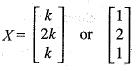Courses

# Test: Linear Algebra - 6

## 20 Questions MCQ Test Topic-wise Tests & Solved Examples for IIT JAM Mathematics | Test: Linear Algebra - 6

Description
This mock test of Test: Linear Algebra - 6 for Mathematics helps you for every Mathematics entrance exam. This contains 20 Multiple Choice Questions for Mathematics Test: Linear Algebra - 6 (mcq) to study with solutions a complete question bank. The solved questions answers in this Test: Linear Algebra - 6 quiz give you a good mix of easy questions and tough questions. Mathematics students definitely take this Test: Linear Algebra - 6 exercise for a better result in the exam. You can find other Test: Linear Algebra - 6 extra questions, long questions & short questions for Mathematics on EduRev as well by searching above.
QUESTION: 1

### Find the value of k for which the following simultaneous equations x + y + z = 3; x + 2y + 3z = 4; x + 4y + kz = 6 will not have a unique solution.

Solution:

We need to find the value of k for which the following simultaneous equations
x + y + z= 3
x + 2y + 3z = 4
x + 4y + kz = 6
will not have a unique solution. This system of equation may be written as,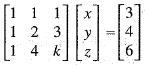Reduce this system of equation to echelon form using the operations
"R2 → R2 - R1" and R3 → R-  R1.
These operations yield -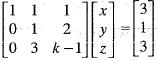and also, R3 → R3 - 3R2 gives,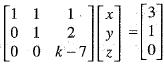also this system of equation has not unique solution if,
k-7 = 0
k = 7

QUESTION: 2

### Solution for the system defined by the set of equations 4y + 3z = 8; 2x - z = 2 and 3x + 2y = 5 is

Solution:

We need to determine the solution for the system of equation
4y+3z= 8
2x - z = 2
3x + 2y= 5
This system of equation may be written in matrix form as,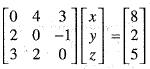Reduce this system of equation to echelon form using the operations
"R2 ⇔ R1". This operations yields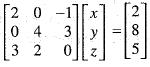and also applying "R→ 2R3 - 3R1" which yields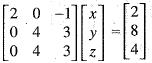again, "R1→ R3 - R2 " we get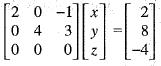Here the coefficient matrix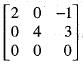and augmented matrix is given by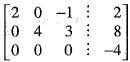Since, the rank of coefficient matrix ≠ the rank of augmented matrix.
Therefore, the solution does not exist.

QUESTION: 3

### Consider the system of simultaneous equation x + 2y + z = 6; 2x+y + 2z = 6; x+y + z = 5 This system has

Solution:

We are given that the system of equation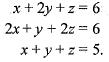This system of equation can be written in augmented matrix as,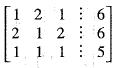Applying the operations "R2 → R2 - 2R1" and "R3 → R3 - R1" these operations yields: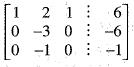also applying R3 → 3R3 - R2, which yields -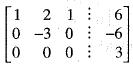Since the rank of coefficient matrix ≠ the rank of augmented matrix.
Therefore, the given system of equations has no solution.

QUESTION: 4

A2x2 matrix which satisfy A2 - A = 0, then

Solution:

We are given that A be any 2 x 2 matrix which satisfy A2 - A = 0
The characteristic equation of the given matrix is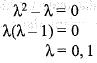There exists distinct eigen values.
Hence, A must be diagonalizable.

QUESTION: 5

A is any n x n matrix with entries equal to 1 then

Solution:

We are given that A is any n x n with entries equal to 1. Suppose, if A is 2 x 2 matrix with entries equal to 1, that is,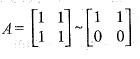multiplicity of 0 is 1.
For 3 x 3 matrix with entries equal to 1,
i.e.,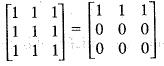multiplicity of 0 is 2.
By continuing this process, for n x n matrix with entries equal to 1, that is,
A =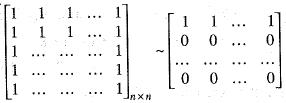multiplicity of 0 is (n - 1).

QUESTION: 6

​Consider the system x + y + z = 0; x - y - z = 0, then the system of equations have

Solution:

We are given that the system of equation,
x + y + z = 0
x - y - z = 0
This system of equations has rank 2 and 3 unknowns,
that is, rank < no. of unknown
Hence, this system of equation has infinitly many solution.

QUESTION: 7

If the linear transformation T : R2 → R3 is such that T(1, 0) = (2,3,1) and T(1,1) = (3, 0,2), then

Solution:

We are given that a linear transformation T : R3→ R3 defined by
T(1, 0) = (2, 3,1)
and T(l, 1) = (3, 0, 2)
We need to find the image of (x, y) under linear transformation T.
Let there exist scalars α and β such that
(x,y) = α(1,0) + β(1, 1)
or equivalently (x, y)= (α + β, β)
Comparing the components on both sides, we get
x = α + β and y = β
Solving for α and β, we get
α = x - y and β = y
Therefore,(x, y)= (x - y) (1, 0) + y(1, 1) Taking the image under linear transformation T. we get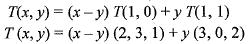implies T(x, y) = (2x + y, 3x - 3y, x+y).

QUESTION: 8

The unique linear transformation T : R→ R2 such that T(1,2) = (2,3) and T(0, 1) = (1,4). Then, the rule for T is.

Solution:

We need to find the linear transformation T : R→ R2 such that
T(1, 2) = (2,3) and T(0, 1) = (1, 4).
Let (x, y) ∈ R2 and α, β are scalars such that
(x,y) = α(1 , 2) + β(0 ,1)
(x,y) = (α , 2α + β)
On comparing the Camponents of coordinates, we get
α = x and y2 = 2α + β
Solving for α and β, we get
α = x and β = y - 2x
Therefore,(x, y) = x (1, 2) + (y - 2x) (0, 1)
Taking the image under linear  transformation T, we get
T(x, y) = T[x(1, 2 ) + (y - 2x) (0,1)]
Using the property of linearity, we get
T(x, y) = xT(1, 2) + (y - 2x) T(0, 1)
Substituting the value of T(1, 2) and T(0, 1), we get
T(x, y) = x(2, 3) + (y - 2x) (1, 4)
= (2x, 3x) + (y - 2x, 4y - 8x)
= (y, 4y-5x)

QUESTION: 9

For a linear transformation T : R10 → R6, the kernal has dimension 5. Then, the dimension of the range of T is

Solution:

We are given a linear transformation T : R10 → R6 and ker T has dimension 5. We need to find the dimension of range T.
using rank nullity theorem, we get dim range T = dim R10 - dim ker T.
= 10 - 5 = 5
Therefore, the dim of range T is 5.

QUESTION: 10

Transformation
(x, y, z) → (x + y, y + z) : R3 → R2 is

Solution:

We are given that a linear transformation T : R3 → R2 defined by T(x, y, z) = (x + y, y + z)
Here, we need to check its linearity and also need to find ker T.
Let (x1, y1, z1) and (x2, y2, z2) be any two vector of R3, Then
T[(x1, y1, z1) + (x2, y2, z2)]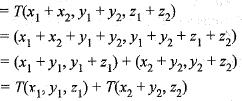Again let α be a scalar number then,
T[α(x,y, z)]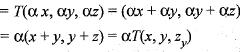Hence, T is a linear.
Let (x, y, z) ∈ ker T, then T(x, y, z) = (0,0)
using the definition of ker T, we get
(x+y,y + z) = (0, 0)
Implies x + y - 0 and
y + z = 0
Implies x = - y and y = - z
Therefore, ker T = {(x, -x, x ) : x e R}
Therefore, dim ker T = 1
Hence, ker T is a proper subspace of R3

QUESTION: 11

Let P2[x] be the vector space of all polynomials over R of degree less than or equal to 2. Let D be the differential operator on P2[x]. Then, matrix of D relative td the basis [x2, 1, x] is equal to

Solution:

Let P2(x) be the vector space of all polynomials over R of degree less than or equal to 2 and D be the differential operator defined on P2[x].
We need to find the matrix of D related to the basis {x3, 1, x} Now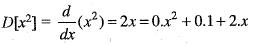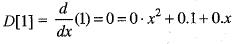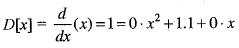Therefore, the matrix of D related to the basis {x2, 1, x} is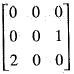QUESTION: 12

If T : V2(R) → V3(R) defined as T(a, b) = (a + b , a-b ,b) is a linear transformation, Then nullity of T is

Solution:

We are given that a linear transformation T: V2(R) → V3(R) defined by
T(a, b) = (a + b , a - b, b).
We need to find the nullity of linear transformation T.
Let (a, b) ∈ ker T, Then
T(a,b)= (0,0,0)
Now using the definition of linear transformation, we get
(a + b, a - b , b) = (0, 0, 0)
Comparing the components of the co-ordinates, we get
a + b = 0, a - b = 0 , b = 0
Solving for a, b, we get
a = 0,6 = 0
Therefore,ker T = {(0, 0)}
Thus, dim (ker T) = 0, that is nullity of linear transformation T is zero.

QUESTION: 13

The transition matrix P from the standard ordered basis to the ordered basis {(1, 1), (-1,0)} is

Solution:

We need to find the transition matrix P from the standard ordered basis to the ordered basis {(1, 1), (-1, 0)}.
We know that two standard ordered basis for R2 is {(1, 0), (0, 1)}.
Therefore, Let there exists scalars α and β such that
(1 ,0 )= α (1, 1) + β (-1,0 )
or equivalently (1,0) = (α - β, α )
Comparing the components of the coordinates, we get α - β = 1, α = 0 Solving for α and β, we get α = 0, β = 1 Therefore, (1, 0) = 0(1, 1) - 1(-1, 0) Again let there be scalars a and P such that
(0 ,1) = α(l, 1) + β ( - l , 0) equivalently(0, 1) = (α - β, α)
Comparing the components of the coordinates, we get α - β = 0, α = 1 Solving for α and β we get
α = 1, β = 1.
Therefore, (0, 1) = 1(1,1) + 1(-1, 0)
By using (1,0) = 0(1, 1) - 1(-1, 0)
and (0,1) = 1(1, 1) + 1 (- 1 ,0 )
The transition matrix is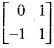QUESTION: 14

Let F be any field and let T be a linear operator on F2 defined by T(a, b) = (a + b, a), then T-1(a, b) is equal to:

Solution:

Let F be any field and let T be a linear operator on F2 defined by
T(a, b) = (a + b, a)
We need to find T-1(a, b).
Let (a, b) ∈ ker T.
Then T(a, b) = (0, 0)
Using the definition of linear transformation, we get
(a + b, a) = (0, 0)
Comparing the components of the coordinates, we get
a + b = 0, a = 0
Solving for α and β, we get a = 0, b = 0.
Therefore,ker T = {(0,0)}
Hence, T is one-one. Since T is a linear transformation on finite dimensional vector space F2. Therefore, T is onto. Hence, T is invertible linear transformation.
Let (x,y) be the image of (a,b) under T-1.
Then (x, y) = T-1(a, b) or equivalently T(x, y) = (a, b)
Using the definition of linear transformation,
we get (x+y,y)= (a,b)
Comparing the components of the coordinates, we get
x + y = a and y = b
Solving for x and y, we get x= a - b , y = b.
Therefore,T-1(a, b) = (a - b, b)

QUESTION: 15

A linear transformation T : R2 → R2 such that T(3, 1) = (2, -4) and T(1, 1) = (0,2). Then, T(7, 8) is

Solution:

We are given that a linear transformation T : R2 → R2
such that T(3, l ) = (2,- 4).
and T(1,1) = (0, 2)
We need to find T(7, 8).
Let there exist scalar α and β such that (7, 8)
= α (3,1) + β(1,1)
or equivalently (7,8) = (3α + β, α + β)
On comparing the components of coordinates, we get
3α + β = 7 and α + β = 8
Solving for α and β, we get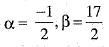Therefore,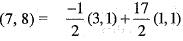Now taking the image under linear transformation T, we get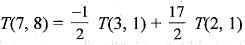Substituting the values of T(3, 1) and T(1, 1), we get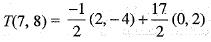= (-1,2) + (0,17) = (-1,19)
Thus, the image of (7,8) under the linear transformation T is (-1, 19).

QUESTION: 16

A is any matrix which satisfy A3 - A2 + A - I = 0 and A3x3, then A4 is

Solution:

We are given that, A be any 3 x 3 matrix which satisfy
A3- A+ A - I = 0
and we need to find A4.
A3- A2+ A - I = 0
or A3 = A 2 - A + I
or A4 = A3 - A2 + A

QUESTION: 17

The eigen values of the matrix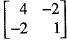are

Solution:

We need to find the eigen values of the matrix A =The characteristics equation of the given matrix A,
|A - λI |= 0
or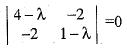or (4 - λ) (1 - λ) - 4 = 0
or λ2 - 5λ = 0
=> λ(λ-5) = 0
=> λ = 0,5
The eigen values of the given matrix is 0 and 5.

QUESTION: 18

Which one of the following is an eigen vector of the matrix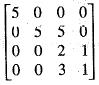Solution:

We need to find the eigen vector of the matrix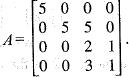The characteristic equation of the given matrix is,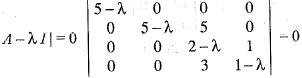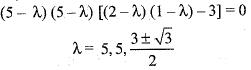Put λ = 5 in equation | A - λI | X = 0 that is,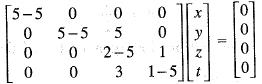or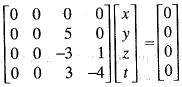5z =0
-3z +1 = 0
3z - 4f = 0
z = 0, t = 0
and also let x = k1, y = k2
Hence, the eigen vector corresponding to the eigen value λ= 5 is,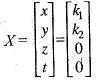Let k1 = 1, and k= -2, then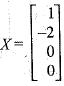QUESTION: 19

The eigen vectors o f the matrix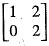are written in the form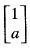and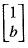.  What is a + b ?

Solution:

We are given that the eigen vectors o f the matrix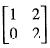are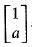and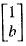. We need to find the value o f a + b. The characteristic equation o f the given matrix is, |A - λI| = 0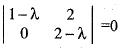or (1 - λ)(2 - λ) = 0 or
λ = 1,2
Put λ = 1 in the equation [ A- λI ] X= 0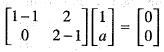or a = 0 and also put X = 2 in the equation [A - λI] X= 0
or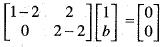or 1+2b = 0
or b = 1/2
Now, a + b = 0 + 1/2
a + b = 1/2

QUESTION: 20

An eigen vector o f A =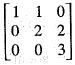is

Solution:

We need to find the eigen vector of the matrix A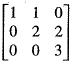The characteristic equation of the given matrix is, |A - λI| = 0
or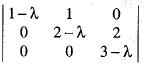= 0
or (1 - λ) (2 - λ,) (3 - λ) = 0
λ = 1,2,3
Suppose, X be the eigen vector corresponding to the eigen value λ = 3.
Hence,  (A - λI) X = 0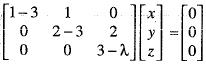or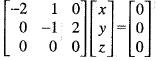or -2x + y = 0
-y + 2z = 0
Let x = k
Hence y = 2k and z = k
Now, the eigen vector is,